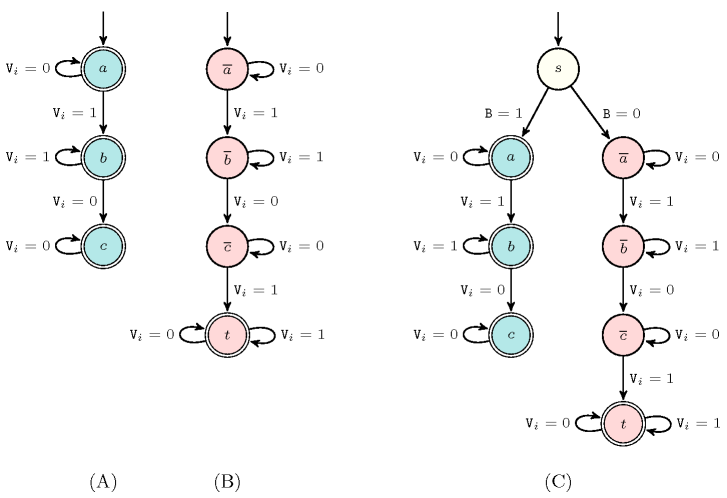### 3.7.208. Reified automaton constraint

A constraint $𝒞\left({V}_{1},{V}_{2},\cdots ,{V}_{n}\right)$ for which the reified version can be mechanically constructed from the finite deterministic automaton ${𝒜}^{𝒞}$ that only accepts the set of solutions of $𝒞$. This is done by deriving from ${𝒜}^{𝒞}$ a so called reified automaton ${𝒜}_{¬𝒞}^{𝒞}$ by:

• First, adding a 0-1 variable $B$ in front of the sequence of variables ${V}_{1},{V}_{2},\cdots ,{V}_{n}$. This new sequence of variables will be passed to the reified automaton ${𝒜}_{¬𝒞}^{𝒞}$.

• Second, constructing from ${𝒜}^{𝒞}$ the automaton ${𝒜}_{¬𝒞}$ that only recognises non-solutions of $𝒞$.

• Third, building from the two automata ${𝒜}^{𝒞}$ and ${𝒜}_{¬𝒞}$ the automaton ${𝒜}_{¬𝒞}^{𝒞}$. This is done by:

1. Creating the initial state $s$ of ${𝒜}_{¬𝒞}^{𝒞}$.

2. Adding a transition labelled by value 1 from $s$ to the initial state of ${𝒜}^{𝒞}$.

3. Adding a transition labelled by value 0 from $s$ to the initial state of ${𝒜}_{¬𝒞}$.

##### Figure 3.7.55. (A) The automaton for recognising the solutions to the $\mathrm{𝚐𝚕𝚘𝚋𝚊𝚕}_\mathrm{𝚌𝚘𝚗𝚝𝚒𝚐𝚞𝚒𝚝𝚢}$ constraint; (B) the automaton for recognising the non-solutions to the $\mathrm{𝚐𝚕𝚘𝚋𝚊𝚕}_\mathrm{𝚌𝚘𝚗𝚝𝚒𝚐𝚞𝚒𝚝𝚢}$ constraint; (C) the automaton for the reified $\mathrm{𝚐𝚕𝚘𝚋𝚊𝚕}_\mathrm{𝚌𝚘𝚗𝚝𝚒𝚐𝚞𝚒𝚝𝚢}$ constraint.Figure 3.7.55 illustrates the construction of a reified automaton in the context of the $\mathrm{𝚐𝚕𝚘𝚋𝚊𝚕}_\mathrm{𝚌𝚘𝚗𝚝𝚒𝚐𝚞𝚒𝚝𝚢}$ constraint. Part (A) recalls the automaton that only recognises the solutions to the $\mathrm{𝚐𝚕𝚘𝚋𝚊𝚕}_\mathrm{𝚌𝚘𝚗𝚝𝚒𝚐𝚞𝚒𝚝𝚢}$ constraint. Assuming the same alphabet $\left\{0,1\right\}$, Part (B) provides the automaton that only recognises the non-solutions to the $\mathrm{𝚐𝚕𝚘𝚋𝚊𝚕}_\mathrm{𝚌𝚘𝚗𝚝𝚒𝚐𝚞𝚒𝚝𝚢}$ constraint. Finally, Part (C) depicts the reified automaton constructed from the two automata given in parts (A) and (B).